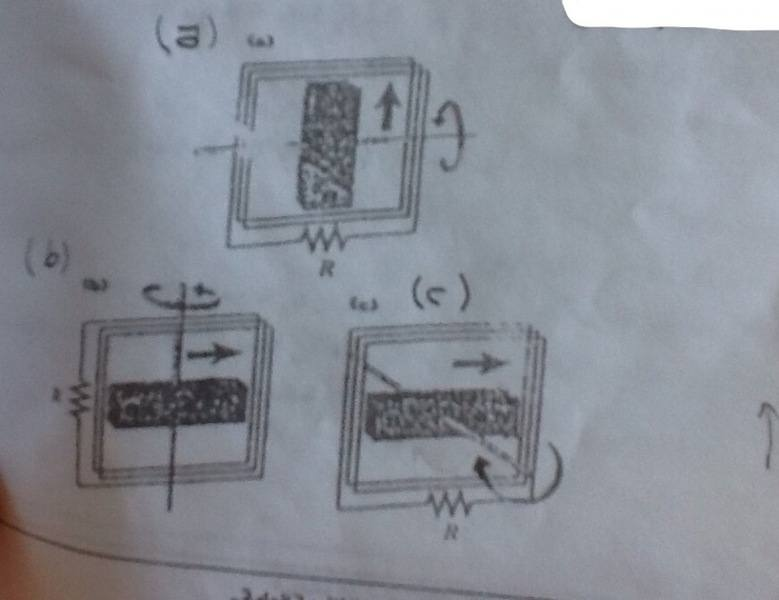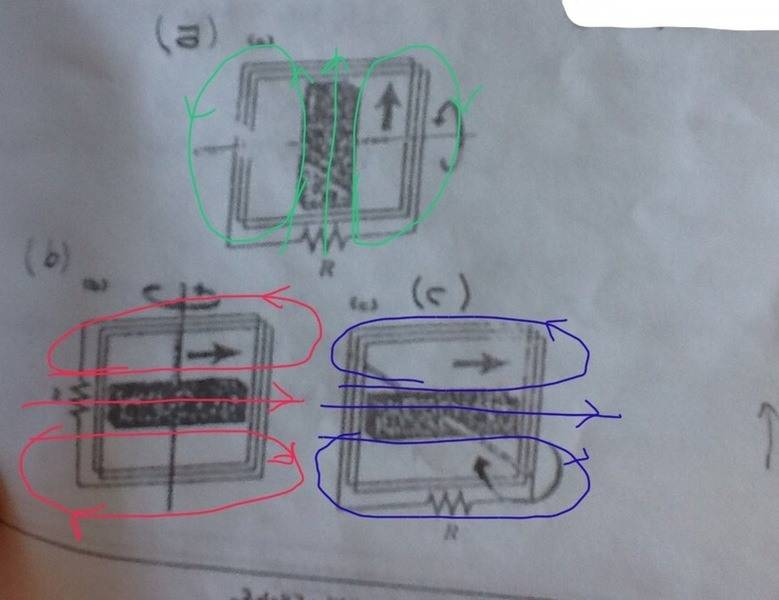# Which drawing represents an AC generator?

## Homework Statement

A magnet bar has been installed in the vertical rotation axis of a coil. Which of these drawings represents an AC generator?2. Relevant rules

Fleming's right-hand rule.

## The Attempt at a Solution

Fleming's right-hand rule assumes that the coil is in motion, but in the drawings the magnet is in motion, and that's what made me not able to know how to apply it correctly here. So could anyone give me some hints, please?
I think both (a) and (b) could be AC generators, but the problem statement says that only one is correct.

## Answers and Replies

Homework Helper
Gold Member
2021 Award
Draw some field lines and see in which drawing(s) they could be in a direction perpendicular to the plane of the loop as the magnet rotates.

David Lewis
I don't see significant difference between (a) and (b).
Also, what is meant by vertical rotation axis?

Draw some field lines and see in which drawing(s) they could be in a direction perpendicular to the plane of the loop as the magnet rotates.
Ok, here is it:And from that figure I would exclude drawing (c), but still (a) and (b) have a chance to be AC generators, right?
I don't see significant difference between (a) and (b).
Also, what is meant by vertical rotation axis?
I agree with you regarding (a) and (b), and also I don't understand what does it meen by saying "vertical rotation axis"!

Homework Helper
Gold Member
The emf should be induced "along" the length of the coil. For this to happen,a component of magnetic field, "linear" velocity (instantaneous) 'v' of the magnet and length of the coil should be perpendicular to each other.

The emf should be induced "along" the length of the coil. For this to happen,a component of magnetic field, "linear" velocity (instantaneous) 'v' of the magnet and length of the coil should be perpendicular to each other.
Ok, I think this happens in both (a) and (b), doesn't it?

Homework Helper
Gold Member
Ok, I think this happens in both (a) and (b), doesn't it?
Looks like it does.
So both a and b can be generators.

Looks like it does.
So both a and b can be generators.
That's what confuses me actually, because the book says only one drawing is correct. Some people told me that may there be something regarding " the vertical rotation axis" which I don't understand, neither I understand what does it mean by "vertical rotation axis".

Homework Helper
Gold Member
That's what confuses me actually, because the book says only one drawing is correct. Some people told me that may there be something regarding " the vertical rotation axis" which I don't understand, neither I understand what does it mean by "vertical rotation axis".
If you just rotated b through 90 degrees CCW, you'll get a (I haven't checked the polarity).
So I think both the options are correct.
They have specified the axis of rotation and direction of rotation of the magnet in each case. So you need not think what vertical axis means here.